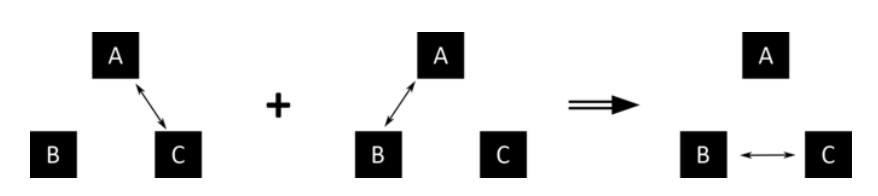Cyber Sale - Only 3 Days Remaining: ALL MCAT Products & Admissions Services

MCAT Content / Energy Changes In Chemical Reactions / Zeroth Law Concept Of Temperature

### Zeroth Law: Concept of Temperature

Topic: Energy Changes In Chemical Reactions

The Zeroth Law of Thermodynamics states that, if system A and system B are in thermal equilibrium with system C, then system A and system B are in thermal equilibrium with each other.

Temperature is a property of matter that describes the thermal energy of a system; in common terms, temperature is used to describe the sensation of  “cool” or “hot”. When a system is said to be in equilibrium, this means that all forces in a system are balanced and are not opposing each other. In the case of thermal equilibrium, a system has an unchanging state of temperature.Referencing the zeroth law, if system A and system B are in thermal equilibrium with system C, this means that the temperature for A and B is the same as C. Hence, the temperature for A and B are the same, which is why they are in thermal equilibrium with each other as well.

Key Points

• The Zeroth Law of Thermodynamics states if systems A and B are in thermal equilibrium with C, then A and B are in thermal equilibrium with each other.

Key Terms

thermal equilibrium: the condition where two systems in contact with each other exchange no energy and have the same temperature

temperature: a measure of the thermal energy in a system

Billing Information